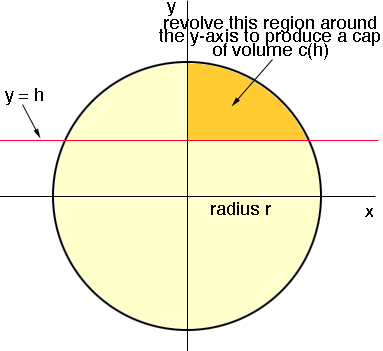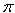SEARCH HOMEMath Central Quandaries & QueriesQuestion from Rory, a student: Cut a given sphere by a plane so that the volumes of the two segments formed shall be in a given ratio. Show that in modern notation, this leads to the cubic equation m(r+x)^2(2r-x)=n(r-x)^2(2r+x) where r is the radius of the sphere, x is the distance of the cutting plane from the centre of the sphere, and m/n < 1 is the given ratio. Can you help me show the solution to this question. Thank you.Hi Rory,

I started with the circle in the plane with centre at the origin and radius r. I then sliced the circle with the line y = h, h > 0. (I am using h where you used x in your expression.) I then took the region inside the circle, above the line y = h and in the first quadrant, and revolved it about the y-axis to get a cap of the sphere.I then used the method of cylindrical shells to find the volume of this cap and called the volume c(h). The volume of the remainder of the sphere is 4/3r3 - c(h). These volumes are to be in the ratio of m to n where n > m so

m/n = c(h)/[4/3r3 - c(h)]

I then simplified this expression and compared it to the expression you sent us, with x replaced by h.

I hope this helps,
PennyMath Central is supported by the University of Regina and The Pacific Institute for the Mathematical Sciences.